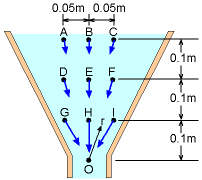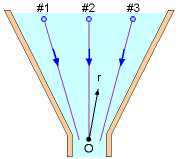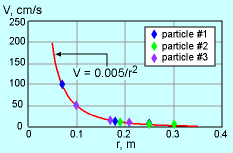Ch 3. Fluid Kinematics Multimedia Engineering Fluids FlowDescriptions Steady &Unsteady Streamlines,Streaklines Velocity &Acceleration IrrotationalFlow
 Chapter 1. Basics 2. Fluid Statics 3. Kinematics 4. Laws (Integral) 5. Laws (Diff.) 6. Modeling/Similitude 7. Inviscid 8. Viscous 9. External Flow 10. Open-Channel Appendix Basic Math Units Basic Equations Water/Air Tables Sections Search eBooks Dynamics Fluids Math Mechanics Statics Thermodynamics Author(s): Chean Chin Ngo Kurt Gramoll ©Kurt GramollFLUID MECHANICS - CASE STUDY SOLUTION

Since it is given that the velocity is inversely proportional to the square of the location r, assume the correlation for velocity has the following form:

V = c/r2

where c is a constant which needs to be determined.Schematic of Experiment Set #1Correlation for Experiment Set #1

For experiment set #1, the locations of the measurement points are first determined (using the Pythagorean theorem):

 Sensor Location A B C Location (r), m 0.304 0.300 0.304 Velocity (V), cm/s 5.2 5.6 5.5 c = Vr2, m3/s 0.00481 0.00504 0.00508 Sensor Location D E F Location (r), m 0.206 0.200 0.206 Velocity (V), cm/s 11.5 12.4 11.7 c = Vr2, m3/s 0.00488 0.00496 0.00497 Sensor Location G H I Location (r), m 0.112 0.100 0.112 Velocity (V), cm/s 40.0 50.5 39.5 c = Vr2, m3/s 0.00502 0.00505 0.00495

By taking the average of the calculated values for the constant c, it is found that c is 0.00497, which is close to 0.005. The Eulerian viewpoint is used in this experiment since the velocities are measured at fixed locations.Schematic of Experiment Set #2Correlation for Experiment Set #2

For experiment set #2:

 Fluid Particle #1 Location (r), m 0.25 0.18 0.07 Velocity (V), cm/s 8.1 15.5 101.8 c = Vr2, m3/s 0.00506 0.00502 0.00499 Fluid Particle #2 Location (r), m 0.30 0.25 0.19 Velocity (V), cm/s 5.7 7.8 14.0 c = Vr2, m3/s 0.00513 0.00488 0.00505 Fluid Particle #3 Location (r), m 0.21 0.17 0.10 Velocity (V), cm/s 11.0 16.9 50.0 c = Vr2, m3/s 0.00485 0.00488 0.00500

The average value for the constant c is 0.00498, which is again close to 0.005. Since the measurements in set #2 are taken by following individual fluid particles, the flow field is determined using the Lagrangian viewpoint.

Practice Homework and Test problems now available in the 'Eng Fluids' mobile app
Includes over 250 problems with complete detailed solutions.
Available now at the Google Play Store and Apple App Store.